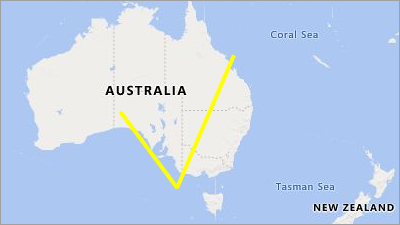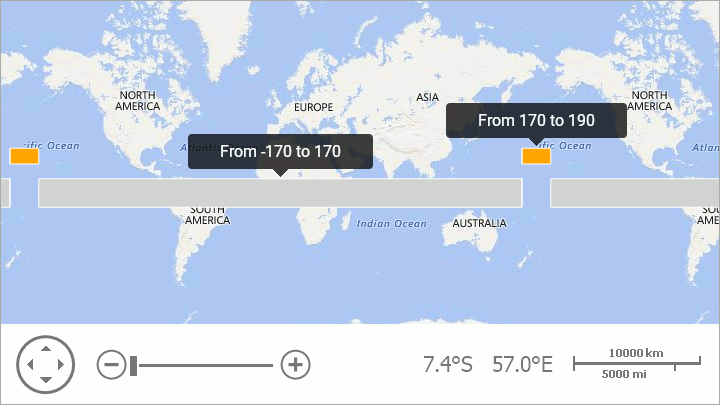20.2
20.1
19.2
19.1
18.2
18.1
17.2
A newer version of this page is available. Switch to the current version.

# MapPolyline Class

The class used to draw a polyline on a map.

Namespace: DevExpress.XtraMap

Assembly: DevExpress.XtraMap.v18.2.dll

## Declaration

``````public class MapPolyline :
MapShape,
IPointContainerCore,
ISupportCoordPoints,
ISupportIntermediatePoints,
IEditableItem``````
``````Public Class MapPolyline
Inherits MapShape
Implements IPointContainerCore,
ISupportCoordPoints,
ISupportIntermediatePoints,
IEditableItem``````

## Related API Members

The following members accept/return MapPolyline objects:

## Remarks

The following image shows an example of a map polyline object.Note that, a map shape should fulfill the following condition to cross the 180th meridian: one or several points' longitudes should exceed the 180 (-180) limit:

``````
MapPolygon polygon = new MapPolygon();
polygon.Points.Add(new GeoPoint(10, -170));
polygon.Points.Add(new GeoPoint(10, 170));
polygon.Points.Add(new GeoPoint(-10, 170));
polygon.Points.Add(new GeoPoint(-10, -170));
vectorItemStorage.Items.Add(polygon);

// Point longitudes enclose the 180 meridian.
MapPolygon polygonCross180 = New MapPolygon();
polygonCross180.Fill = Color.Orange;
polygonCross180.Points.Add(new GeoPoint(30, 190));
polygonCross180.Points.Add(new GeoPoint(30, 170));
polygonCross180.Points.Add(new GeoPoint(20, 170));
polygonCross180.Points.Add(new GeoPoint(20, 190));
vectorItemStorage.Items.Add(polygon180);
``````

The code above produces the following image:## Examples

##### NOTE

A complete sample project is available at https://github.com/DevExpress-Examples/how-to-create-different-map-shapes-t139819

``````var polyline = new MapPolyline() { StrokeWidth = 4, Stroke = Color.Yellow };
polyline.Points.AddRange(new GeoPoint[] {
new GeoPoint(-29,130),
new GeoPoint(-40,140),
new GeoPoint(-20,150)
});
ItemStorage.Items.Add(polyline);
``````

See Also